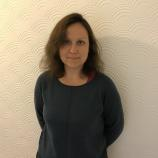Article

# Construction of Artificial Point Sources for a Linear Wave Equation in Unknown Medium

## Details

Citation

Kirpichnikova A, Korpela J, Lassas M & Oksanen L (2021) Construction of Artificial Point Sources for a Linear Wave Equation in Unknown Medium. SIAM Journal on Control and Optimization, 59 (5), pp. 3737-3761. https://doi.org/10.1137/20M136904X

Abstract
We study the wave equation on a bounded domain of \$\mathbb R^m\$ and on a compact Riemannian manifold \$M\$ with boundary. We assume that the coefficients of the wave equation are unknown but that we are given the hyperbolic Neumann-to-Dirichlet map \$\Lambda\$ that corresponds to the physical measurements on the boundary. Using the knowledge of \$\Lambda\$ we construct a sequence of Neumann boundary values so that at a time \$T\$ the corresponding waves converge to zero while the time derivative of the waves converge to a delta distribution. The limit of such waves can be considered as a wave produced by an artificial point source. The convergence of the wave takes place in the function spaces naturally related to the energy of the wave. We apply the results for inverse problems and demonstrate the focusing of the waves numerically in the 1-dimensional case.

Keywords
Focusing of waves; Neumann-to-Dirichlet map; Inverse problems

Journal
SIAM Journal on Control and Optimization: Volume 59, Issue 5

Status Published Engineering and Physical Sciences Research Council 31/12/2021 18/10/2021 10/08/2021 http://hdl.handle.net/1893/33222 0363-0129 1095-7138

## PeopleDr Anna Kirpichnikova

Lecturer, Mathematics